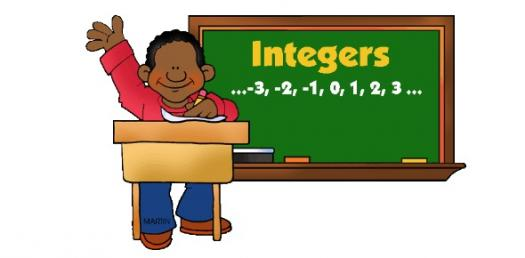# Integers Quiz Questions And Answers

5 Questions | Attempts: 1362SettingsThis quiz will assess student's prior knowledge of integers.

• 1.
A negative plus a negative equals
• A.

A negative

• B.

A positive

• C.

Zero

• 2.
A positive plus a positive equals
• A.

A negative

• B.

A positive

• C.

Zero

• 3.
When you add integers with different signs, you must subtract and take the sign of the largest number.
• A.

True

• B.

False

• 4.
When you multiply or divide integers with the same sign, the answer is always negative.
• A.

True

• B.

False

• 5.
-8 x 5 =
• A.

40

• B.

-40

• C.

-3

## Related TopicsBack to top
×

Wait!
Here's an interesting quiz for you.